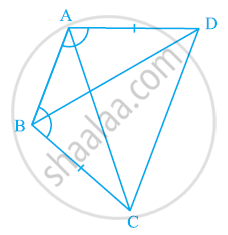# ABCD is a quadrilateral in which AD = BC and ∠DAB = ∠CBA (See the given figure). Prove that - Mathematics

ABCD is a quadrilateral in which AD = BC and ∠DAB = ∠CBA (See the given figure). Prove that

(i) ΔABD ≅ ΔBAC

(ii) BD = AC

(iii) ∠ABD = ∠BAC.#### Solution

In ΔABD and ΔBAC,

∠DAB = ∠CBA (Given)

AB = BA (Common)

∴ ΔABD ≅ ΔBAC (By SAS congruence rule)

∴ BD = AC (By CPCT)

And, ∠ABD = ∠BAC (By CPCT)

Concept: Criteria for Congruence of Triangles
Is there an error in this question or solution?

#### APPEARS IN

NCERT Class 9 Maths
Chapter 7 Triangles
Exercise 7.1 | Q 2 | Page 119